This section of the guide describes custom shaders and shows you how to implement them within Unity.

When creating objects in Unity, it helps the user’s eye if the object looks as real as possible. Shaders have an important part to play in making the object look real. This is because the shaders hold the mathematical calculations that render the pixels.

Unity 5 and higher includes a Physically Based Shading (PBS) model which is incorporated in the standard shader. PBS simulates the interactions between material and light. PBS also provides a high level of realism and makes it possible to achieve a consistent look under different lighting conditions.

Note: You can easily use PBS with the standard shader. If you create your own material it defaults to using the standard shader.

There are several other built-in shaders including the standard shader. The following image shows how you can see all the available built-in shaders: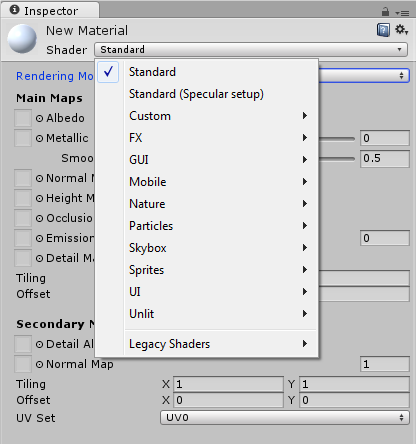There are many effects that cannot be achieved by using existing shaders. For example, standard shaders cannot implement reflections based on local cubemaps. For more information, see Local cubemap rendering techniques.

In Unity there are three main ways of customizing shaders:

• Shader Graph (URP and HDRP only) allows a shader to be graphically created, putting together the wanted effects see, the blog post Introduction to Shader Graph for more information.
• Surface shaders (standard Render Pipeline only) are commonly used when lights and shadows affect the shaders. Unity does the work related to the lighting model for you, enabling you to write more compact shaders.
• Vertex and fragment shaders are the most flexible shaders, but you must implement everything. Unity contains a powerful shading and material language called ShaderLab, which focuses on more than vertex and fragment shaders. But vertex and fragment shaders are the main programmable part of the graphics pipeline where shading is done.

The following code shows a simple vertex and fragment shader that contains most of the elements required in a vertex or fragment shader:

Note: The example shader is written in Cg. Unity also supports the HLSL language for shader snippets. The Cg program snippets are written between `CGPROGRAM` and `ENDCG`.

```Shader "Custom/ctTextured"
{
Properties
{
_AmbientColor ("Ambient Color", Color) = (0.2,0.2,0.2,1.0)
_MainTex ("Base (RGB)", 2D) = "white" {}
}
{
Pass
{
CGPROGRAM
#pragma target 3.0
#pragma glsl
#pragma vertex vert
#pragma fragment frag
#include "UnityCG.cginc"
// User-specified uniforms
uniform float4 _AmbientColor;
uniform sampler2D _MainTex;
struct vertexInput
{
float4 vertex : POSITION;
float4 texCoord : TEXCOORD0;
};
struct vertexOutput
{
float4 pos : SV_POSITION;
float4 tex : TEXCOORD0;
};
vertexOutput vert(vertexInput input)
{
vertexOutput output;
output.tex = input.texCoord;
output.pos = mul(UNITY_MATRIX_MVP, input.vertex);
return output;
}
float4 frag(vertexOutput input) : COLOR
{
float4 texColor = tex2D(_MainTex, input.tex);
return _AmbientColor + texColor;
}
ENDCG
}
}
Fallback "Diffuse"
}
```

The first line in the code is `Shader: Custom/cTextured`, which is the path/name of the shader. The path defines the category where the shader is displayed in the drop-down menu when you are setting a material. The shader from the example is displayed under the category of custom shaders in the drop-down menu.

The `Properties{}` block lists the shader parameters that are visible in the inspector and what parameters you can interact with.

Each shader in Unity contains of a list of `SubShaders`. When Unity renders a mesh, it looks for a shader to use and selects the first `SubShader` that can run on the graphics card. This way shaders are executed correctly on different graphics cards that support different shader models. Executing shaders this way is important. This is because GPU hardware and Application Programming Interfaces (APIs) are constantly evolving. For example, you can write your main shader targeting an Arm Mali Midgard GPU to use the latest features of OpenGL ES 3.0. Then in a separate `subshader`, write a replacement shader for graphics cards supporting OpenGL ES 2.0 and earlier.

The `Pass` block causes the geometry of an object to be rendered once. A shader can contain one or more passes. You can use multiple passes on old hardware, or to achieve special effects, for example hardware that only supports an early OpenGL ES version might need multiple passes to achieve an effect that you can do in one pass in new hardware.

If Unity cannot find a `SubShader` in the body of the shader that can render the geometry correctly, it rolls back to another shader that is defined after the Fallback statement. In our example, the `SubShader` is the `Diffuse` built-in shader.

#### Compilation directives

The compilation directives indicate the shader functions that need to be compiled. You pass compilation directives as `#pragma` statements.

Each compilation directive must contain at least the directives to compile the vertex and the fragment shader, for example: `#pragma vertex name`, `#pragma fragment name`.

By default, Unity compiles shaders into Shader Model 2.0. However, the directive #pragma target enables shaders to be compiled into other capability levels. Shaders being compiled into other capability levels are useful as Shader model 2.0 has a low instruction limit. Therefore, if the shader becomes large you get an error of the following type:

```Shader error in 'Custom/MyShader': Arithmetic instruction limit of 64 exceeded; 83 arithmetic instructions needed to compile program;
```

If you get the preceding error message, you must change from Shader Model 2.0 to Shader Model 3.0 by adding `#pragma target 3.0`. This is because the Shader model 3.0 has a much higher instruction limit.

Passing several varying variables from vertex shader to fragment shader might cause the following error:

```Shader error in 'Custom/MyShader': Too many interpolators used (maybe you want #pragma glsl?) at line 75.
```

To resolve the preceding error, add the compilation directive `#pragma glsl`. This directive converts Cg or HLSL code into GLSL.

Unity supports several rendering APIs like `vulkan`, `gles`, `gles3`, OpenGL, `d3d11`, `d3d11_9x`, Xbox one, and PS4. By default, all these shaders are compiled to the platform. However, you can explicitly limit this number using the #pragma only_renderers, followed by the render APIs that you want, leaving a blank space between them.

Note: If you are targeting mobile devices only, limit shader compilations to `vulkan`, `gles`, and `gles3`. You must also add the `opengl` and `d3d11` renderers that are used by the Unity Editor, as shown in the following example:

```#pragma only_renderers vulkan gles gles3 [opengl, d3d11]
```

#### Includes

In a shader include files can be added. Unity makes available include files that give predefined variables and helper functions. Available includes can be found in `C:\Program Files\Unity\Hub\Editor\<version>\Editor\Data\CGIncludes`. For example, in the include `UnityCG.cginc` there are several useful helper functions and macros that are used in many standard shaders. To use these functions or macros, declare the includes in your shader.

`UnityShaderVariables.cginc` is included automatically in Unity and contains many built-in variables that are available to shaders in the include. This means that `UnityShaderVariables.cginc` does not need to be declared. Several useful transformation matrices and magnitudes are directly available in the shaders. It is important to know if they are available in the shaders to avoid doing unnecessary work. For example, if you need them, there may be includes that provide you with the following:

• A matrix to the shader
• Camera position
• Projection parameters
• Light parameters

Sometimes it improves performance to execute an operation in the CPU. Then pass the result to the GPU instead of executing it in the vertex shader for every vertex, multiplication of matrix uniforms is an example of this. For this reason, Unity makes available several compound matrices as built-in uniforms. Some of the important Unity shader built-in values are shown in the following table:

 Built-in Uniform Description `UNITY_MATRIX_V` Current view matrix `UNITY_MATRIX_P` Current projection matrix `Object2World` Inverse of current world matrix `_World2Object` Current projection matrix `UNITY_MATRIX_VP` Current view * projection matrix `UNITY_MATRIX_MV` Current model * view matrix `UNITY_MATRIX_MVP` Current model * view * projection matrix `UNITY_MATRIX_IT_MV` Invert transpose of current model * view matrix `_WorldSpaceCameraPos` Camera position in world space `_ProjectionParams` Near and far planes and 1/farPlane as components of a vector `_Time` Current time and fractions in a vector (t/20, t, t*2, t*3)

#### OpenGL ES 3.0 and Vulkan graphics pipelines

OpenGL ES and Vulkan are two different APIs. However, their graphics pipelines are essentially the same, therefore, the vertex and fragment shaders sit in similar places.

Note: This section of the guide does not compare OpenGL ES and Vulkan. Instead, itdescribes the generalized graphics API pipeline. Therefore, if you would like more information see Vulkan: Migrating from OpenGL ES

The following image shows a schematic view of the OpenGL ES 3.0 graphic pipeline flow:Let us look at what is happening at each stage of the graphic pipeline flow:

• In the primitives’ stage, the pipeline operates on the geometric primitives that are described by vertices, points, lines, and polygons.
• The vertex shader implements a general-purpose programmable method for operating on vertices. The vertex shader transforms and lights vertices.
• In primitive assembly, the vertices are assembled into geometric primitives. The resulting primitives are clipped to a clipping volume and sent to the rasterizer.
• Output values from the vertex shader are calculated for every generated fragment. This process is known as interpolation. During rasterization, the primitives are converted into a set of two-dimensional fragments that are then sent to the fragment shader.
• Transform feedback enables selective writing to an output buffer from the vertex shader and is later sent back to the vertex shader. This feature is not exposed by Unity, but it is used internally to, for example, optimize the skinning of characters.
• The fragment shader implements a general-purpose programmable method for operating on fragments before they are sent to the next stage.
• In per-fragment operations, several functions and tests are applied on each fragment:
• Pixel ownership test
• Scissor test
• Stencil
• Depth tests
• Blending
• Dithering
Applying the preceding functions and tests in the per-fragment stage means that either:
• Fragment color, depth, or stencil value must write to the frame buffer in screen coordinates.
• The frame buffer discards the fragment.

The vertex shader example that is shown in Shader structure runs once for every vertex of the geometry. The vertex shader transforms the 3D position of each vertex to the projected 2D position in screen space. Then the vertex shader calculates the depth value for the Z-buffer.

The transformed position is expected in the output of the vertex shader. If the vertex shader does not return a value, the console displays the following error:

```Shader error in 'Custom/ctTextured': '' : function does not return a value: vert at line 36
```

In the Shader structure example, the vertex shader receives the vertex coordinates in local space and the texture coordinates. Vertex coordinates are transformed from local to screen space using the Model View Projection matrix: `UNITY_MATRIX_MVP`. The Model View Projection matrix is a Unity built-in value, as shown in the following code:

```output.pos = mul(UNITY_MATRIX_MVP, input.vertex);
```

Note: Texture coordinates are passed to the fragment shaders as a varying variable, but this does not mean that they are not transformed.

Normal vectors are transformed from object space to world space in a different manner. To guarantee that the normal is still normal to the triangle after a non-uniform scaling operation, it must be multiplied by the transpose of the inverse of the transformation matrix. To apply the transpose operation, you flip the order of factors in the multiplication. The inverse of the local to world matrix is the built-in `World2Object` Unity matrix. This matrix is a 4x4 matrix so you must build a four-component vector from the three-component normal input vector, as shown in the following code:

```float4 normalWorld = mul(float4(input.normal, 0.0), _World2Object);
```

When building the four-component vector, you add a zero as the fourth component. Adding zero as the fourth component is necessary to handle vector transformation correctly in four-dimensional space. In contrast, for co-ordinates the fourth component of the vector must be one.

If normals are already supplied in world coordinates, you can skip the process of normal transformation, which saves work in the vertex shader. This optimization cannot be used if the object mesh could potentially be handled by any Unity built-in shader. This is because then normals are expected in object coordinates.

Most graphic effects are implemented in the fragment shader, but some effects can be done in the vertex shader. For example, vertex displacement mapping, also known as displacement mapping, is a well-known technique that enables you to deform a polygonal mesh using a texture to add surface detail, height maps in terrain generation are an example of this. To access this texture in the vertex shader, also known as displacement map, you must add the pragma directive `#pragma target 3.0`. This is because the displacement map is only available in Shader Model 3.0. According to the Shader Model 3.0, at least four texture units must be accessible inside the vertex shader. However, if you force the editor to use the OpenGL renderer, then `#pragma glsl` must be added to the shader. If this directive is not declared, the following error message appears:

```Shader error in 'Custom/ctTextured': function "tex2D" not supported in this profile (maybe you want #pragma glsl?) at line 57
```

In the vertex shader, you can also animate vertices using procedural animation techniques. You can use the time variable in shaders enabling modification of the vertex coordinates as a function of time. Mesh skinning is another type of functionality implemented in the vertex shader. Unity uses mesh skinning to animate the vertices of the meshes associated with character skeletons.

Structures define the input and output of the vertex shader. In the input structure of the Shader structure example, you declare only the vertex attributes position and texture coordinates.

You can define more attributes as input, for example:

• A second set of texture coordinates
• Normal vectors in object coordinates
• Colors
• Tangents

The preceding list can be defined using the following semantics:

```struct vertexInput
{
float4 vertex : POSITION;
float4 tangent : TANGENT;
float3 normal : NORMAL;
float4 texcoord : TEXCOORD0;
float4 texcoord1 : TEXCOORD1;
fixed4 color : COLOR;
};
```

Note: A semantic is a string attached to a shader input or output that provides information about the use of a parameter. You must specify a semantic for all variables passed between shader stages.

If you use the incorrect semantics like `float3 tangent2 : TANGENTIAL`, you get an error of the following type:

```Shader error in 'Custom/ctTextured': unknown semantics "TANGENTIAL" specified for "tangent2" at line 32
```

For optimum performance, only specify the parameters in the input structure that you require. Unity has some predefined input structures for the most common cases of input parameter combinations in the `UnityCG.cginc` include file. For example, `appdata_base`, `appdata_tan` and `appdata_full`. The previous vertex input structure example corresponds to `appdata_full`, where you are not required to declare the structure, only declare the include file.

#### Vertex shader output and varyings

Vertex shader output is defined in an output structure that must contain the vertex transformed coordinates.

The following example shows the output structure which lists the semantics supported by Unity, but you can add other magnitudes:

```struct vertexOutput
{
float4 pos : SV_POSITION;
float4 tex : TEXCOORD0;
float4 texSpecular : TEXCOORD1;
float3 vertexInWorld : TEXCOORD2;
float3 viewDirInWorld : TEXCOORD3;
float3 normalInWorld : TEXCOORD4;
float3 vertexToLightInWorld : TEXCOORD5;
float4 vertexInScreenCoords : TEXCOORD6;
};
```

In the preceding example, the transformed vertex coordinates are defined with the semantic `SV_POSITION`. Two textures, several vectors, and coordinates in different spaces calling the semantic `TEXCOORDn` are also passed to the fragment shader.

`TEXCOORD0` is typically reserved for UVs and `TEXCOORD1` for lightmap UVs, but you can send anything from `TEXCOORD0` to `TEXCOORD7` to the fragment shader. It is important to notice that each interpolator, that is, each semantic, can only process a maximum of four floats, putting larger variables like matrices into multiple interpolators. Therefore, if you define a matrix to be passed as a varying: `float4x4 myMatrix : TEXCOORD2`, Unity uses the interpolators from `TEXCOORD2` to `TEXCOORD5`.

Everything that you send from the vertex shader to the fragment shader is linearly interpolated by default. The rasterizer is the stage of the graphics pipeline that turns vertex data into pixels ready to feed into the fragment shader (a pixel shader). Every triangle is defined by two vertices – which we can label `V1`, `V2` and `V3`. The rasterizer calculates pixel co-ordinates and data values for the pixel through a linear interpolation of the vertices using barycentric co-ordinates - λ1, λ2 and λ3. This is shown in the following image:The following image shows `V1` being fully red, `V2` fully green and `V3` fully blue and the rasterizer linearly interpolating the color values between them to get the color for each pixel in between: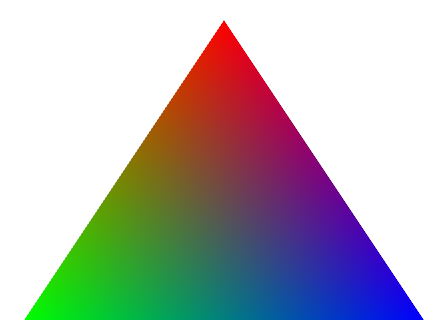The same interpolation is applied to any varying that is passed from the vertex to the fragment shader. The int interpolation in the rasterizer is a very powerful tool because there is a hardware linear interpolator. For example, if you want to apply a color as a function of the distance to a point C, you pass the coordinates of C to the vertex shader. The vertex shader then calculates the squared distance from the vertex to C and passes that magnitude to the fragment shader. The distance value is automatically interpolated for you in every pixel of every triangle.

Values are linearly interpolated, so it is possible to perform per-vertex computations and reuse them in the fragment shader. In other words, a value that is linearly interpolated in the fragment shader can be calculated in the vertex shader instead. Calculating the value in the vertex shader can provide a substantial performance boost. This is because the vertex shader runs on a much smaller data set than the fragment shader.

Note: You must be careful with the use of varyings, especially in mobile phones where performance and memory bandwidth consumption are critical to the success of many games. The more varyings there are, the more bandwidths that are used on vertex accesses and fragment shader varying reads. Therefore, aim for a reasonable balance of data versus quality when using varyings.

The fragment shader is after the primitive rasterization on the graphics pipeline stage, as shown in the following image:For each sample of the pixels that is covered by a primitive, a fragment is generated. The fragment shader code is executed for every generated fragment. There are many more fragments than vertices, so you must be careful about the number of operations that are performed in the fragment shader.

In the fragment shader you can access the fragment co-ordinates in 3D screen space. Similarly, all other values that have been interpolated from per-vertex values output by the vertex shader are available in 3D screen space.

In the shader example that is shown in Shader structure, the fragment shader receives the interpolated texture coordinates from the vertex shader. The fragment shader performs a texture lookup to obtain the color at these coordinates. The fragment shader then combines this color with the ambient color to produce the final output color. From the declaration of the fragment shader `float4 frag(vertexOutput input) : COLOR` it is clear that the shader is expected to ouput the fragment color. The fragment shader is where you calculate the look you want the geometry to have. The fragment shader achieves this by assigning the correct color to each fragment.

Any data that is declared as a uniform in the Pass block in the Shader structure code example is available to both the vertex shader and the fragment shaders.

A uniform is like a global constant variable because it cannot be modified inside the shader.

You can supply this uniform to the shader in the following ways:

• Using the `Properties` block
• Programmatically from a script

The `Properties` block enables you to define uniforms interactively in the Inspector. Any variable that is declared in the Properties block appears in the material inspector that is listed with the variable name.

The following code shows the `Properties` block of the shader example that is associated with the material `ctSphereMat`:

```Properties
{
_AmbientColor ("Ambient Color", Color) = (0.2,0.2,0.2,1.0)
_MainTex ("Base (RGB)", 2D) = "white" {}
}
```

The variables `_AmbientColor` and `_MainTex` that are declared in the Properties block with the names `Ambient Color` and `Base (RGB)` appear in the Material Inspector with those names.

The following image shows the `Properties` of the Material Inspector: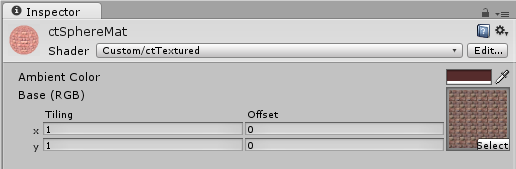Passing data to the shader through the `Properties` block is very useful. Passing data this way is especially useful when you are in the development stage of the shader. This is because you can change the data interactively and see the effect at runtime.

You can put the following types of variables in the `Properties` block:

• Float
• Color
• Texture 2D
• Cubemap
• Rectangle
• Vector

Consider a situation in which data is required from a previous calculation or data is required to be passed at specific point in time. In these situations, the `Properties` block is not a useful way of passing data.

Another way to pass data to the shaders is programmatically from a script. The material class exposes several methods that you can use to pass data associated with a material to a shader. The following table lists the most common methods:

 Method `SetColor (propertyName: string, color: Color);` `SetFloat (propertyName: string, value: float);` `SetInt (propertyName: string, value: int);` `SetMatrix (propertyName: string, matrix: Matrix4x4);` `SetVector (propertyName: string, vector: Vector4);` `SetTexture (propertyName: string, texture: Texture);`

In the following code `shwMats` contains a list of materials. The code shows how the materials send matrices and textures to the shader. Immediately before the main camera renders the scene, a secondary camera shwCam renders the shadows to a texture which combines with the main camera render pass:

```// Called before object is rendered.
public void OnWillRenderObject()
{
// Perform different checks.
...
// Set up shadows camera shwCam.
...
// Pass matrices to the shader
for(int i = 0; i < shwMats.Count; i++)
{
shwMats[i].SetMatrix("_ShwCamProjMat", shwCam.projectionMatrix);
shwMats[i].SetMatrix("_ShwCamViewMat", shwCam.transform.worldToLocalMatrix);
}
shwCam.Render();
for(int i = 0; i < shwMats.Count; i++)
{
}
}
```

For the shadow texture projection process, the vertices must be transformed in a convenient manner. Therefore, the following passes to the shader:

• Shadow camera projection matrix: `shwCam.projectionMatrix`
• World to local transformation matrix: `shwCam.transform.worldToLocalMatrix`
• The rendered shadow texture: `m_ShadowsTexture`

These values are available in the shader as uniforms with the names `_ShwCamProjMat`, `_ShwCamViewMat` and `m_ShadowsTexture`.

In Unity, it is not possible to debug shaders in the same way that you debug with traditional code. You can, however, use the output of the fragment shader to visualize the values that you want to debug. You can then interpret the image produced.

The following image shows the output of the shader `ctReflLocalCubemap.shader` applied to the reflective surface of the floor: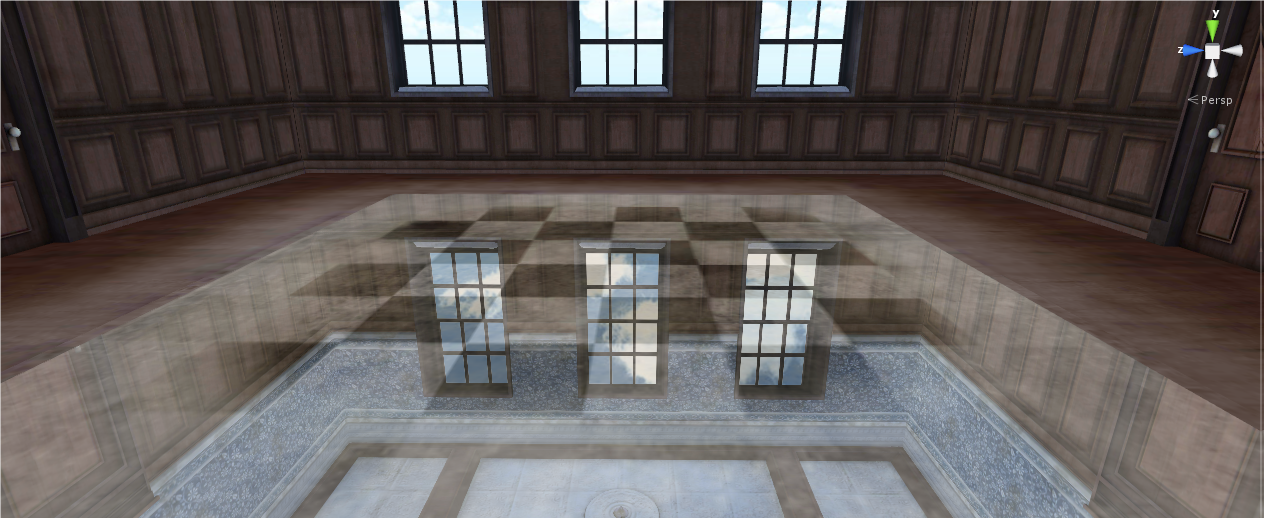In the fragment shader code, you can replace the output color with the normalized local corrected reflection vector, as follows:

```return float4(normalize(localCorrReflDirWS), 1.0);
```

Instead of the reflected image, the output it visualizes the components of the reflected vector normalized as colors.

The reddish color zone in the floor indicates that the reflected vector has a strong X component. This means that the reflected vector is mostly orienting towards the X Axis. The red area of the image shows that the reflection comes from that direction, that is, from the windowed wall.

The blue zone indicates a predominance of reflected vectors that are oriented to the Z axis, which is shown as the reflection from the right wall.

In the black zone, the vectors are mainly orienting to –Z, but the colors can only have positive components. This is because the negative components clamp to 0.

The following image shows the result of replacing the output color of the fragment with the normalized local reflected vector: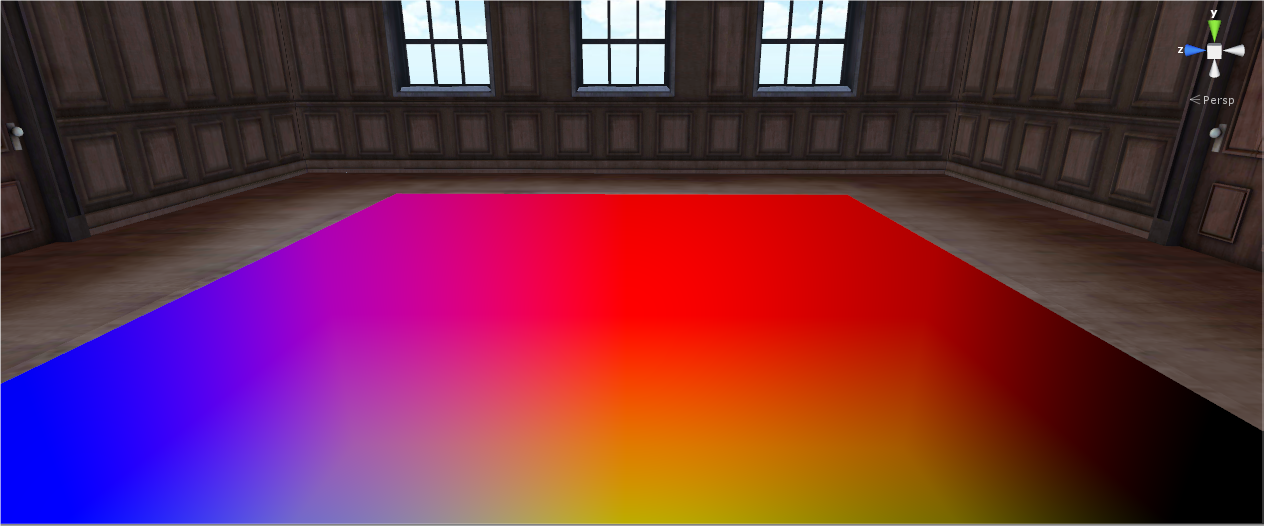Initially it is difficult to interpret the meaning of the colors while debugging. Therefore, you should try to focus on a single-color component. For example, you can return only the Y component of the normalized local corrected reflected vector, as shown in the following code:

```float3 normLocalCorrReflDirWS = normalize(localCorrReflDirWS);
return float4(0, normLocalCorrReflDirWS.y, 0, 1);
```

In the preceding code, the output is only the reflections that are coming mainly from the roof above the camera. This is the part of the room that is oriented to the Y axis. The reflections from the walls of the room are coming from the X, Z, and –Z directions, so they are rendered in black.

The following image shows shader debugging with a single color: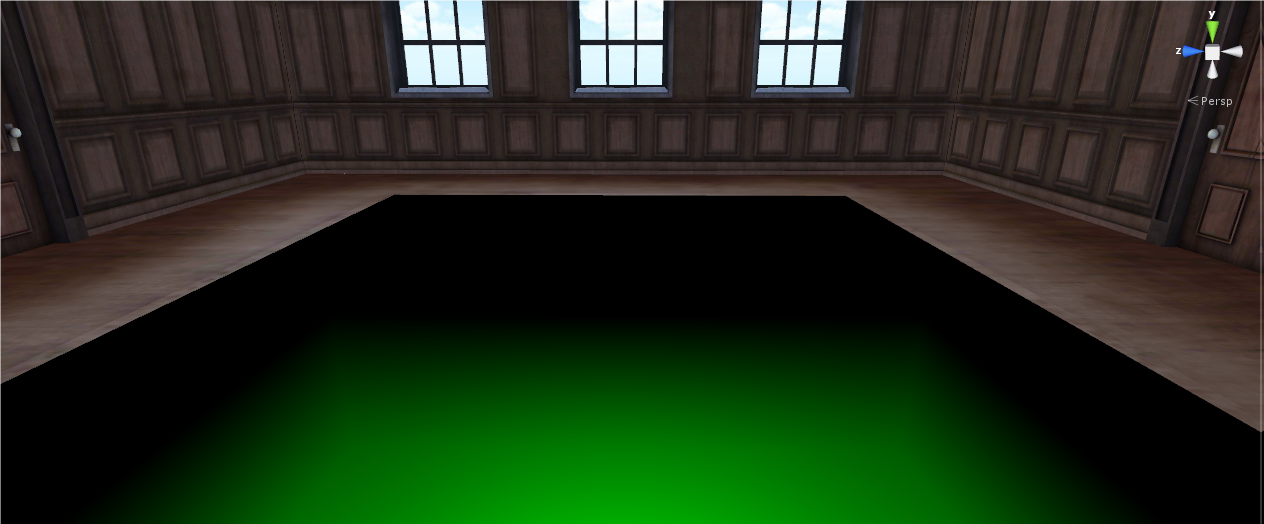Note: You must check that the magnitude you are debugging with color is between zero and one. This is because any other value is automatically clamped. Any negative value is assigned zero and any value greater than one is assigned one.